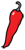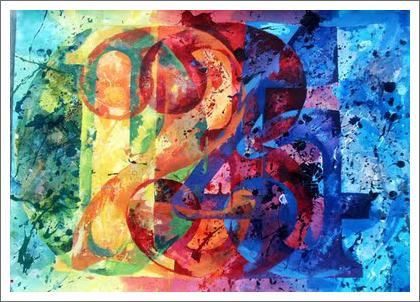The Grand Locus / Life for statistical sciences

the Blog

## Infinite expectationsIn the first days of my PhD, I sincerely believed that there was a chance I would find a cure against cancer. As this possibility became more and more remote, and as it became obvious that my work would not mark a paradigm shift, I became envious of those few people who did change the face of science during their PhD. One of them is Andrey Kolmogorov, whose PhD work was nothing less than the modern theory of probability. His most famous result was the strong law of large numbers, which essentially says that random fluctuations become infinitesimal on average. Simply put, if you flip a fair coin a large number of times, the frequency that ‘tails’ turn up will be very close to the expected value 1/2.

### The chaos of large numbersMost fascinating about the strong law of large numbers is that it is a theorem, which means that it comes with hypotheses that do not always hold. There are cases that repeating a random experiment a very large number of times does not guarantee that you will get closer to the expected value — I wrote the gory detail on Cross Validated, for those interested...

## Unpredictable?

What if I told you to choose a card at random? Simply choose one from a standard deck of 52 cards, think of a card, do not draw one from a real deck... Make sure you have one in mind before you read on.

### The illusion

Assuming that you have a card in mind, I bet you chose the Ace of Spades. Of course, I don't know the card you were thinking of, but that is the one I bet on. Every textbook on probability says that I have a 1/52 chance of having guessed right. Actually, that is not quite true... About one out of 4 readers will choose the Ace of Spades, and one out of seven will choose the Queen of Hearts, as shown by a study of the researcher in psychology of magic Jay Olson and his collaborators.

In this experiment, is the choice of a card purely random? And what does random mean anyway? Even if purely random is not properly defined, most would agree that it means no information at all, or completely unpredictable. The outcome of the experiment above is clearly not what you would call purely random. But...

## The chaos and the doubt

Probability is said to be born of the correspondence between Pierre de Fermat and Blaise Pascal, some time in the middle of the 17th century. Somewhat surprisingly, many texts retrace the history of the concept up until the 20th century; yet it has gone through major transformations since then. Probability always describes what we don't know about the world, but the focus has shifted from the world to what we don't know.

Henri Poincaré investigates in Science et Méthode (1908) why chance would ever happen in a deterministic world. Like most of his contemporaries, Poincaré believed in absolute determinism, there is no phenomenon without a cause, even though our limited minds may fail to understand or see it. He distinguishes two flavors of randomness, of which he gives examples.

If a cone stands on its point we know that it will fall but we do not know which way (...) A very small cause, which escapes us, determines a considerable effect that we can not but see, and then we say that this effect is due to chance.

And a little bit later he continues.

How do we represent a container filled with gas? Countless molecules...

## Poetry and optimalityClaude Shannon was the hell of a scientist. His work in the field of information theory, (and in particular his famous noisy channel coding theorem) shaped the modern technological landscape, but also gave profound insight in the theory of probabilities.

In my previous post on statistical independence, I argued that causality is not a statistical concept, because all that matters to statistics is the sampling of events, which may not reflect their occurrence. On the other hand, the concept of information fits gracefully in the general framework of Bayesian probability and gives a key interpretation of statistical independence.

Shannon defines the information of an event with probability $Prob(A)$ as $-\log P(A)$. For years, this definition baffled me for its simplicity and its abstruseness. Yet it is actually intuitive. Let us call $\Omega$ the system under study and $\omega$ its state. You can think of $\Omega$ as a set of possible messages and of $\omega$ as the true message transmitted over a channel, or (if you are Bayesian) of $\Omega$ as a parameter set and $\omega$ as the true value of the parameter. We have total information about the system if we know $\omega$. If instead, all...

## The fallacy of (in)dependenceIn the post Why p-values are crap I argued that independence is a key assumption of statistical testing and that it almost never holds in practical cases, explaining how p-values can be insanely low even in the absence of effect. However, I did not explain how to test independence. As a matter of fact I did not even define independence because the concept is much more complex than it seems.

Apart from the singular case of Bayes theorem, which I referred to in my previous post, the many conflicts of probability theory have been settled by axiomatization. Instead of saying what probabilities are, the current definition says what properties they have. Likewise, independence is defined axiomatically by saying that events $A$ and $B$ are independent if $P(A \cap B) = P(A)P(B)$, or in English, if the probability of observing both is the product of their individual probabilities. Not very intuitive, but if we recall that $P(A|B) = P(A \cap B)/P(B)$, we see that an alternative formulation of the independence of $A$ and $B$ is $P(A | B) = P(A)$. In other words, if $A$ and $B$ are independent, observing...

## The reverend’s gambitTwo years after the death of Reverend Thomas Bayes in 1761, the famous theorem that bears his name was published. The legend has it he felt the devilish nature of his result and was too afraid of the reaction of the Church to publish it during his lifetime. Two hundred and fifty years later, the theorem still sparkles debate, but among statisticians.

Bayes theorem is the object of the academic fight between the so-called frequentist and Bayesian schools. Actually, more shocking than this profound disagreement is the overall tolerance for both points of view. After all, Bayes theorem is a theorem. Mathematicians do not argue over the Pythagorean Theorem: either there is a proof or there isn’t. There is no arguing about that.

So what’s wrong with Bayes theorem? Well, it’s the hypotheses. According to the frequentist, the theorem is right, it is just not applicable in the conditions used by the Bayesian. In short, the theorem says that if $A$ and $B$ are events, the probability of $A$ given that $B$ occurred is $P(A|B) = P(B|A) P(A)/P(B)$. The focus of the fight is the term $(P(B... ## Why p-values are crapI remember my statistics classes as a student. To do a t-test we had to carry out a series of tedious calculations and in the end look up the value in a table. Making those tables cost an enormous amount of sweat from talented statisticians, so you had only three tables, for three significance levels: 5%, 1% and 0.1%. This explains the common way to indicate significance in scientific papers, with one (*), two (**) or three (***) stars. Today, students use computers to do the calcultations so the star notation probably appears as a mysterious folklore and the idea of using a statistical table is properly unthinkable. And this is a good thing because computing those t statistics by hand was a pain. But statistical softwares also paved the way for the invasion of p-values in the scientific literature. To understand what is wrong with p-values, we will need to go deeper in the theory of statistical testing, so let us review the basic principles. Every statistical test consists of a null hypothesis, a test statistic (a score) and a decision rule — plus the often forgotten alternative hypothesis. A statistical test is an investigation protocol to... ## Drunk man walkingLotteries fascinate the human mind. In the The Lottery in Babylon, Jorge Luis Borges describes a city where the lottery takes a progressively dominant part in people’s life, to the extent that every decision, even life and death, becomes subject to the lottery. In this story, Borges brings us face to face with the discomfort that the concept of randomness creates in our mind. Paradoxes are like lighthouses, they indicate a dangerous reef, where the human mind can easily slip and fall into madness, but they also show us the way to greater understanding. One of the oldest paradoxes of probability theory is the so called Saint Petersburg paradox, which has been teasing statisticians since 1713. Imagine I offered you to play the following game: if you toss ‘tails’, you gain$1, and as long as you toss ‘tails’, you double your gains. The first ‘heads’ ends the spree and determines how much you gain. So you could gain $0,$1, $2,$4, \$8... with probability 1/2, 1/4, 1/8, 1/16, 1/32 etc. What is the fair price I can ask you to play the Saint Petersburg lottery?

Probability theory says that the...